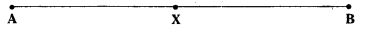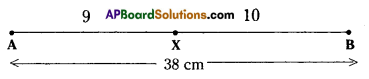AP State Syllabus AP Board 7th Class Maths Solutions Chapter 6 Ratio – Applications Ex 1 Textbook Questions and Answers.

## AP State Syllabus 7th Class Maths Solutions 6th Lesson Ratio – Applications Exercise 1Question 1.
What is the ratio of ₹ 100 and ₹ 10? Express your answer in the simplest form.
Solution:
Ratio of ₹ 100 and ₹ 10 = 100 : 10 = $$\frac{100}{10}=\frac{10}{1}$$ = 10 : 1
Simplest form = 10 : 1.

Question 2.
Sudha has ₹ 5. Money with Radha is 3 times the money with Sudha. How much money does Radha have?
(i) What is the ratio of Radha’s money and Sudha’s money?
(ii) What is the ratio of Sudha’s money and Radha’s money?
Solution:
Money with Sud ha = 5
∴ MoneywltbRadha = 3 times Sudha
= 3 × ₹5 = ₹ 15

i) Radha : Sudha 15 : 5
$$\frac{15}{5}=\frac{3}{1}$$
= 3 : 1

ii) Sudha:Radha = ₹ 5 : ₹ 15
= 5 : 15
= $$\frac{5}{15}$$
= 1 : 3Question 3.
Divide 96 chocolates between Raju and Ravi in the ratio 5 : 7
Solution:
Given ratio = 5 : 7
Sum of the terms of the ratio = 5 + 7 = 12
TotaL chocolates = 96
∴ Rajus share $$\frac{5}{12}$$ x 96 = 40
Ravis share $$\frac{7}{12}$$ x 96 = 56

Question 4.
The length of a line segment AB is 38 cm. A point X on it divides it in the ratio 9: 10. Find the lengths of the line segments AX and XB.Solution:Given ratio AX : XF = 9 : 10
Sum of the terms of the ratio = 9 + 10 = 19
Total length of the line segment = 38 cm
∴ $$\overline{\mathrm{AX}}=\frac{9}{19}$$ × 38 = 18cm
$$\overline{\mathrm{XB}}=\frac{10}{19}$$ × 38 = 20cmQuestion 5.
A sum of ₹ 160,000 is divided in the ratio of 3 : 5. What is the smaller share’?
Solution:
Given that the sum is divided in the ratio = 3: 5
Sum divided = ₹ 1,60,000
Sum of the terms of the ratio 3 + 5 = 8
∴ Smaller share = $$\frac{3}{8}$$ × 1,60.000 = ₹ 60,000

Question 6.
To make green paint, a painter mixes yellow paint and blue paint in the ratio of 3 :2. If he
used twelve liters of yellow paint, how much blue paint did he use ?
Solution:
Ratio of yellow paint and blue paint = 3: 2
Quantity of yellow paint = 3 parts = 12 litres
Quantity of blue paint = 2 parts = $$\frac{2}{3}$$ × 12 = 8 litresQuestion 7.
A rectangle measures 40 cm at its length and 20 cm at its width. Find the ratio of the length
to the width.
Solution:
Length of the rectangle = 40cm
Width of the rectangle = 20cm
Ratio of length and width = 40 cm : 20 cm
= $$\frac{40}{20}=\frac{2}{1}$$ = 2 : 1

Question 8.
The speed of a Garden-Snail is 50 meters per hour and that of the Cheetah is 120 kilometers per hour. Find the ratio of the speeds.
Solution:
Speed of the Garden Snell = 50 m/hour
= $$\frac{50}{1000}$$ kmph
= $$\frac{1}{20}$$ kmph
Speed of the Cheetah = 120 kmph
Ratio of their speeds = $$\frac{1}{20}$$ : 120
= $$\frac{20}{20}$$ : 20 x 120
= 1 : 2400Question 9.
Find (i) The ratio of boys and girls in your class.
(ii) The ratio of number of doors and number of windows of your classroom.
(ii) The ratio of number of text books and number of note books with you